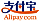Buchcover von Weyl'S Lemma (Laplace Equation)
Buchtitel:

Weyl'S Lemma (Laplace Equation)

Laplace'S Equation, Mollifier, Smooth

PlaisPublishing (19.04.2012 )gutscheinfähig
ISBN-13:

978-613-8-74001-8

ISBN-10:
6138740017
EAN:
9786138740018
Buchsprache:
Englisch
Klappentext:
Please note that the content of this book primarily consists of articles available from Wikipedia or other free sources online. In mathematics, Weyl's lemma is a result that provides a "very weak" form of the Laplace equation. It is named after the German mathematician Hermann Weyl.Weyl's lemma can be proved by convolving the function u with an appropriate mollifier, and then showing that the resulting function satisfies the mean value property, which is equivalent to being harmonic. The nature of the mollifer chosen means that, except on a set of measure zero, the function u is equal to its own mollifier.Weyl's lemma follows from more general results concerning regularity properties of elliptic operators. For example, one way to see why the lemma holds is to note that elliptic operators do not shrink singular support and that 0 has no singular support.
Verlag:
PlaisPublishing
Webseite:
http://www.betascript-publishing.com
Herausgegeben von:
Jody Cletus
Seitenanzahl:
72
Veröffentlicht am:
19.04.2012
Lagerbestand:
Lieferbar
Kategorie:
Mathematik
Preis:
29,00 €
Stichworte:
function, Statement, Equation, Lemma, laplace, S, Weak, Weyl, Weyl', (Laplace, Equation)Kategorien